# ISEE Upper Level Quantitative : Prisms

## Example Questions

### Example Question #1 : How To Find The Surface Area Of A Prismis a positive number. Which is the greater quantity?

(A) The surface area of a rectangular prism with length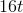, width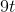, and height(B) The surface area of a rectangular prism with length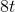, width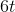, and height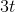.

(A) is greater

(B) is greater

It is impossible to determine which is greater from the information given

(A) and (B) are equal

(A) is greater

Explanation:

The surface area of a rectangular prism can be determined using the formula: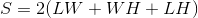Using substitutions, the surface areas of the prisms can be found as follows:

The prism in (A):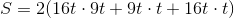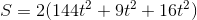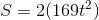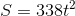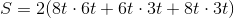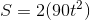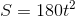Regardless of the value of,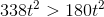- that is, the first prism has the greater surface area. (A) is greater.

### Example Question #1 : How To Find The Length Of An Edge

A cube has sidelength one and one-half feet; a rectangular prism of equal volume has length 27 inches and height 9 inches. Give the width of the prism in inches.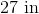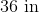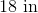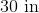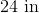Explanation:

One and one half feet is equal to eighteen inches, so the volume of the cube, in cubic inches, is the cube of this, or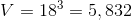cubic inches.

The volume of a rectangular prism is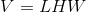Since its volume is the same as that of the cube, and its length and height are 27 and 9 inches, respectively,  we can rewrite this as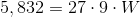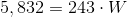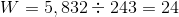The width is 24 inches.

### Example Question #3 : Solid Geometry

A cube has sidelength one and one-half feet; a rectangular prism of equal surface area has length 27 inches and height 9 inches. Give the width of the prism in inches.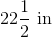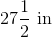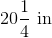Explanation:

One and one half feet is equal to eighteen inches, so the surface area of the cube, in square inches, is six times the square of this, or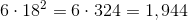square inches.

The surface area of a rectangular prism is determined by the formula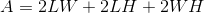.

So, with substitutiton, we can find the width: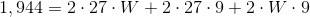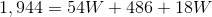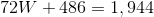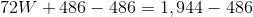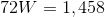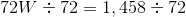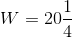inches

### Example Question #1 : Solid Geometry

A rectangular prism has volume one cubic foot; its length and width are, respectively, 9 inches andinches. Which of the following represents the height of the prism in inches?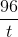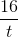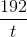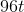Explanation:

The volume of a rectangular prism is the product of its length, its width, and its height. The prism's volume of one cubic foot is equal to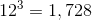cubic inches.

Therefore,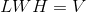can be rewritten as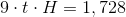.

We can solve for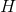as follows: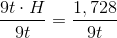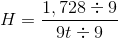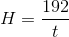### Example Question #1 : Find The Volume Of A Right Rectangular Prism With Fractional Edge Lengths: Ccss.Math.Content.6.G.A.2

A large crate in the shape of a rectangular prism has dimensions 5 feet by 4 feet by 12 feet. Give its volume in cubic yards.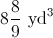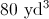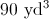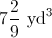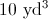Explanation:

Divide each dimension by 3 to convert feet to yards, then multiply the three dimensions together: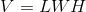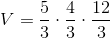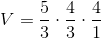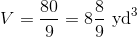### Example Question #61 : Geometry

Which is the greater quantity?

(A) The volume of a rectangular solid ten inches by twenty inches by fifteen inches

(B) The volume of a cube with sidelength sixteen inches

It is impossible to determine which is greater from the information given

(A) and (B) are equal

(A) is greater

(B) is greater

(B) is greater

Explanation:

The volume of a rectangular solid ten inches by twenty inches by fifteen inches is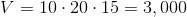cubic inches.

The volume of a cube with sidelength 13 inches is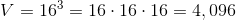cubic inches.

This makes (B) greater

### All ISEE Upper Level Quantitative Resources